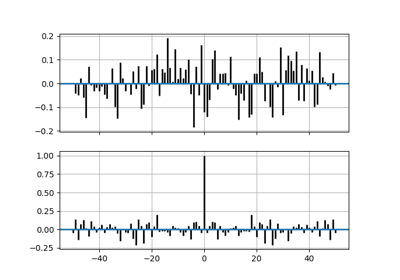/Matplotlib 3.1

# matplotlib.pyplot.xcorr

matplotlib.pyplot.xcorr(x, y, normed=True, detrend=<function detrend_none at 0x7f8092177c80>, usevlines=True, maxlags=10, *, data=None, **kwargs) [source]

Plot the cross correlation between x and y.

The correlation with lag k is defined as $$\sum_n x[n+k] \cdot y^*[n]$$, where $$y^*$$ is the complex conjugate of $$y$$.

Parameters: x : array-like of length n y : array-like of length n detrend : callable, optional, default: mlab.detrend_none x and y are detrended by the detrend callable. This must be a function x = detrend(x) accepting and returning an numpy.array. Default is no normalization. normed : bool, optional, default: True If True, input vectors are normalised to unit length. usevlines : bool, optional, default: True Determines the plot style. If True, vertical lines are plotted from 0 to the xcorr value using Axes.vlines. Additionally, a horizontal line is plotted at y=0 using Axes.axhline. If False, markers are plotted at the xcorr values using Axes.plot. maxlags : int, optional, default: 10 Number of lags to show. If None, will return all 2 * len(x) - 1 lags. lags : array (length 2*maxlags+1) The lag vector. c : array (length 2*maxlags+1) The auto correlation vector. line : LineCollection or Line2D Artist added to the axes of the correlation: LineCollection if usevlines is True. Line2D if usevlines is False. b : Line2D or None Horizontal line at 0 if usevlines is True None usevlines is False. linestyle : Line2D property, optional The linestyle for plotting the data points. Only used if usevlines is False. marker : str, optional, default: 'o' The marker for plotting the data points. Only used if usevlines is False.

#### Notes

The cross correlation is performed with numpy.correlate() with mode = "full".

Note

In addition to the above described arguments, this function can take a data keyword argument. If such a data argument is given, the following arguments are replaced by data[<arg>]:

• All arguments with the following names: 'x', 'y'.

Objects passed as data must support item access (data[<arg>]) and membership test (<arg> in data).

## Examples using matplotlib.pyplot.xcorrCross- and Auto-Correlation Demo